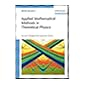Normal view

# Applied mathematical methods in theoretical physics / Michio Masujima.

Material type:TextLanguage: English Publisher: Weinheim : Wiley-VCH, c2009Edition: 2nd, enl. and improved edDescription: xx, 578 p. : ill. ; 25 cmISBN: 9783527409365; 352740936XDDC classification: 530.15 LOC classification: QC19.6 | .M37 2009Online resources: WorldCat details | Ebook Fulltext
Contents:
Function spaces, linear operators, and Green's functions -- Integral equations and Green's functions -- Integral equations of the Volterra type -- Integral equations of the Fredholm type -- Hilbert-Schmidt theory of symmetric kernel -- Singular integral equations of the Cauchy type -- Wiener-Hopf method and Wiener-Hopt integral equation -- Nonlinear integral equations -- Calculus of variations : fundamentals -- Calculus of variations : applications.
Summary: This new edition of the handbook provides all there is to know about functional analysis, integral equations and calculus of variations in one handy volume, written for the specific needs of Read more..
Tags from this library: No tags from this library for this title.Average rating: 0.0 (0 votes)
Item type Current location Collection Call number Copy number Status Date due Barcode Item holdsE-Book
530.15 MAA 2009 (Browse shelf) Not for loanText
Reserve Section
Non-fiction 530.15 MAA 2009 (Browse shelf) C-1 Not For Loan 23580Text
Circulation Section
Non-fiction 530.15 MAA 2009 (Browse shelf) C-2 Available 23728Text
Non-fiction 530.15 MAA 2009 (Browse shelf) C-3 Available 23729Text
Circulation Section
Non-fiction 530.15 MAA 2009 (Browse shelf) C-4 Available 23730
Total holds: 0
##### Browsing EWU Library shelves, Shelving location: Circulation Section Close shelf browser
 No cover image available No cover image available530.143 RAI 2009 Introduction to quantum field theory / 530.143 RAI 2009 Introduction to quantum field theory / 530.15 MAA 2009 Applied mathematical methods in theoretical physics / 530.15 MAA 2009 Applied mathematical methods in theoretical physics / 530.15 RAM 2003 Mathematical physics / 530.15 RAM 2003 Mathematical physics / 530.15 RAM 2003 Mathematical physics /

Includes bibliographical references (p. 567-572) and index.

Function spaces, linear operators, and Green's functions -- Integral equations and Green's functions -- Integral equations of the Volterra type -- Integral equations of the Fredholm type -- Hilbert-Schmidt theory of symmetric kernel -- Singular integral equations of the Cauchy type -- Wiener-Hopf method and Wiener-Hopt integral equation -- Nonlinear integral equations -- Calculus of variations : fundamentals -- Calculus of variations : applications.

This new edition of the handbook provides all there is to know about functional analysis, integral equations and calculus of variations in one handy volume, written for the specific needs of Read more..

Electronics & Communications Engineering

There are no comments for this item.